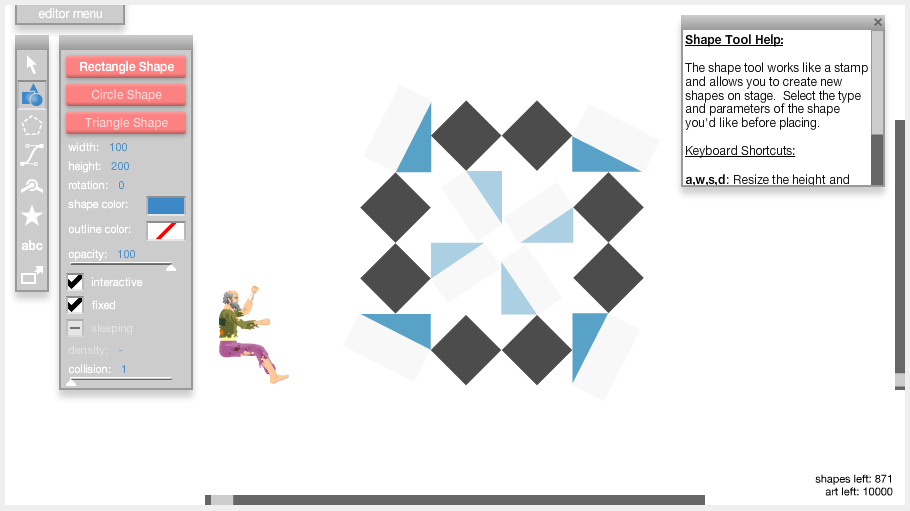# Identicon Gravity Animation

Thursday 5th January 2017

I created a small Easter egg for the homepage of my site: if you click the identicon, it will collapse!Believe it or not, I created this simple animation using Happy Wheels. It's obviously not a proper animation tool, but the level editor allows for very simple and easy 2D physics simulations. I simply recreated my identicon using shapes. The levelXML for the level that I created is available at the bottom of the page.All of the shapes in the level are sleeping at first in order to give time for the camera to move into its resting position. During this time, an invisible ball is falling from the very top of the level area, taking roughly 10 seconds to land on the shapes and trigger the physics.

After recording the animation, I used OpenShot to export the video as an image sequence. Then imported all of those images into GIMP, cropped and adjusted the image and exported as an interpolated GIF.

The GIF was originally a non-looping GIF, however this did not work very well due to browser image caching. Once the GIF had stopped playing, refreshing the page would not cause the browser to replay the GIF from the beginning. Instead, it would just display the final frame of the GIF. In order to combat this, I am now using a non-looping GIF, but the final frame has an extremely long delay. This gives the impression that the GIF has stopped playing, whereas it is really just waiting to display the next frame. If you watch it long enough, the GIF will loop after around 15 minutes.

In order to implement this on my site without using JavaScript or PHP, I used a nifty CSS trick that I found on StackExchange. The trick uses a HTML form checkbox. A different background image is set for each state (checked or unchecked) of the checkbox/label combo, causing the image to change when the checkbox is toggled. This is transparent to the user, since all they can see is a normal image. I ended up changing the checkbox to a single radio button in order to prevent the image from changing multiple times.

I have used a similar animation technique previously in order to create a "Steam for Linux" animation, as seen here.

LevelXML:

```<levelXML>
<info v="1.87" x="244.5" y="9741.7" c="1" f="f" h="t" bg="0" bgc="16316664" e="1"/>
<shapes>
<sh t="0" p0="482.55" p1="9553" p2="50" p3="50" p4="45" p5="f" p6="t" p7="1" p8="5000268" p9="-1" p10="100" p11="2"/>
<sh t="0" p0="553.15" p1="9553.1" p2="50" p3="50" p4="45" p5="f" p6="t" p7="1" p8="5000268" p9="-1" p10="100" p11="2"/>
<sh t="0" p0="624.85" p1="9624.65" p2="50" p3="50" p4="45" p5="f" p6="t" p7="1" p8="5000268" p9="-1" p10="100" p11="2"/>
<sh t="0" p0="624.75" p1="9695.2" p2="50" p3="50" p4="45" p5="f" p6="t" p7="1" p8="5000268" p9="-1" p10="100" p11="2"/>
<sh t="0" p0="553.15" p1="9767.6" p2="50" p3="50" p4="45" p5="f" p6="t" p7="1" p8="5000268" p9="-1" p10="100" p11="2"/>
<sh t="0" p0="482.35" p1="9767.45" p2="50" p3="50" p4="45" p5="f" p6="t" p7="1" p8="5000268" p9="-1" p10="100" p11="2"/>
<sh t="0" p0="411.7" p1="9695.8" p2="50" p3="50" p4="45" p5="f" p6="t" p7="1" p8="5000268" p9="-1" p10="100" p11="2"/>
<sh t="0" p0="411.7" p1="9624.95" p2="50" p3="50" p4="45" p5="f" p6="t" p7="1" p8="5000268" p9="-1" p10="100" p11="2"/>
<sh t="0" p0="531.65" p1="9815.65" p2="590" p3="26" p4="0" p5="t" p6="f" p7="1" p8="-1" p9="-1" p10="100" p11="1"/>
<sh t="1" p0="76.45" p1="139.35" p2="5" p3="5" p4="0" p5="f" p6="f" p7="1" p8="-1" p9="-1" p10="100" p11="1" p12="0"/>
<sh t="0" p0="293.35" p1="184.4" p2="448" p3="5" p4="10" p5="t" p6="f" p7="1" p8="5874375" p9="-1" p10="100" p11="1"/>
<sh t="0" p0="523.3" p1="248.05" p2="5" p3="100" p4="0" p5="t" p6="f" p7="1" p8="5874375" p9="-1" p10="100" p11="1"/>
<sh t="0" p0="249.6" p1="9787.3" p2="30" p3="25" p4="0" p5="t" p6="f" p7="1" p8="-1" p9="-1" p10="100" p11="1"/>
</shapes>
<groups>
<g x="413.9" y="9545.75" r="0" ox="-443.7" oy="-5060.7" s="t" f="f" o="100" im="f" fr="f">
<sh t="0" p0="459.3" p1="5069.2" p2="36" p3="71" p4="0" p5="f" p6="t" p7="1" p8="5874375" p9="-1" p10="100" p11="2"/>
<sh t="0" p0="443.7" p1="5060.7" p2="36" p3="80" p4="27" p5="f" p6="t" p7="1" p8="16316664" p9="-1" p10="100" p11="2"/>
</g>
<g x="632.5" y="9555.75" r="90" ox="-443.7" oy="-5060.7" s="t" f="f" o="100" im="f" fr="f">
<sh t="0" p0="459.3" p1="5069.2" p2="36" p3="71" p4="0" p5="f" p6="t" p7="1" p8="5874375" p9="-1" p10="100" p11="2"/>
<sh t="0" p0="443.7" p1="5060.7" p2="36" p3="80" p4="27" p5="f" p6="t" p7="1" p8="16316664" p9="-1" p10="100" p11="2"/>
</g>
<g x="622.25" y="9774.7" r="180" ox="-443.7" oy="-5060.7" s="t" f="f" o="100" im="f" fr="f">
<sh t="0" p0="459.3" p1="5069.2" p2="36" p3="71" p4="0" p5="f" p6="t" p7="1" p8="5874375" p9="-1" p10="100" p11="2"/>
<sh t="0" p0="443.7" p1="5060.7" p2="36" p3="80" p4="27" p5="f" p6="t" p7="1" p8="16316664" p9="-1" p10="100" p11="2"/>
</g>
<g x="487.95" y="9623.45" r="0" ox="-262.4" oy="-9510" s="t" f="f" o="100" im="f" fr="f">
<sh t="0" p0="274.65" p1="9501.55" p2="36" p3="53" p4="0" p5="f" p6="t" p7="1" p8="11325667" p9="-1" p10="100" p11="2"/>
<sh t="0" p0="262.4" p1="9510" p2="30" p3="65" p4="-34" p5="f" p6="t" p7="1" p8="16316664" p9="-1" p10="100" p11="2"/>
</g>
<g x="554.6" y="9629.9" r="90" ox="-262.4" oy="-9510" s="t" f="f" o="100" im="f" fr="f">
<sh t="0" p0="274.65" p1="9501.55" p2="36" p3="53" p4="0" p5="f" p6="t" p7="1" p8="11325667" p9="-1" p10="100" p11="2"/>
<sh t="0" p0="262.4" p1="9510" p2="30" p3="65" p4="-34" p5="f" p6="t" p7="1" p8="16316664" p9="-1" p10="100" p11="2"/>
</g>
<g x="547.85" y="9697.05" r="180" ox="-262.4" oy="-9510" s="t" f="f" o="100" im="f" fr="f">
<sh t="0" p0="274.65" p1="9501.55" p2="36" p3="53" p4="0" p5="f" p6="t" p7="1" p8="11325667" p9="-1" p10="100" p11="2"/>
<sh t="0" p0="262.4" p1="9510" p2="30" p3="65" p4="-34" p5="f" p6="t" p7="1" p8="16316664" p9="-1" p10="100" p11="2"/>
</g>
<g x="482.25" y="9690.45" r="-90" ox="-262.4" oy="-9510" s="t" f="f" o="100" im="f" fr="f">
<sh t="0" p0="274.65" p1="9501.55" p2="36" p3="53" p4="0" p5="f" p6="t" p7="1" p8="11325667" p9="-1" p10="100" p11="2"/>
<sh t="0" p0="262.4" p1="9510" p2="30" p3="65" p4="-34" p5="f" p6="t" p7="1" p8="16316664" p9="-1" p10="100" p11="2"/>
</g>
<g x="403.2" y="9765" r="0" ox="-403.2" oy="-9765" s="t" f="f" o="100" im="f" fr="f">
<sh t="0" p0="411.6" p1="9749.4" p2="36" p3="71" p4="-90" p5="f" p6="t" p7="1" p8="5874375" p9="-1" p10="100" p11="2"/>
<sh t="0" p0="403.1" p1="9765" p2="36" p3="80" p4="-63" p5="f" p6="t" p7="1" p8="16316664" p9="-1" p10="100" p11="2"/>
</g>
</groups>
</levelXML>```